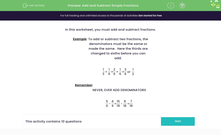# Add and Subtract Simple Fractions

In this worksheet, students will improve their skills at adding and subtracting simple fractions.Key stage:  KS 3

Curriculum topic:   Number

Curriculum subtopic:   Use Four Operations for All Numbers

Popular topics:   Fractions worksheets, Percentages worksheets

Difficulty level:#### Worksheet Overview

In this activity, we will add and subtract fractions.

Example

To add or subtract two fractions, the denominators (bottom numbers) must be the same.

If they are not the same, we must first make them the same.  We can do this by finding a common multiple of both denominators.

If you look below, we can see a common multiple of  3 and 6 is 6.

We must remember to always apply the same rule to the numerator and the denominator.

We will multiply the denominator by 2 to get 6, so we must also multiply the numerator by 2, which gives us 2/6 (have a look below) which we can now add to 1/6.

1/3 + 1/6 = 2/6 + 1/6 = 3/6 or 1/2

Remember that we never add denominators!

Let's get started on the questions now.

### What is EdPlace?

We're your National Curriculum aligned online education content provider helping each child succeed in English, maths and science from year 1 to GCSE. With an EdPlace account you’ll be able to track and measure progress, helping each child achieve their best. We build confidence and attainment by personalising each child’s learning at a level that suits them.

Get started